# Frequency swept signals¶

This page demonstrates two functions in scipy.signal for generating frequency-swept signals: chirp and sweep_poly.

Some of these require SciPy 0.8.

To run the code samples, you will need the following imports:

In :
import numpy as np
from scipy.signal import chirp, sweep_poly


## Linear Chirp¶

Sample code:

In :
t = np.linspace(0, 10, 5001)
w = chirp(t, f0=12.5, f1=2.5, t1=10, method='linear')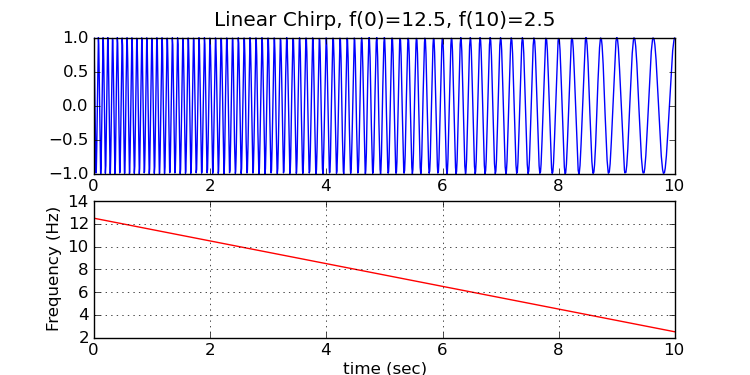Sample code:

In :
t = np.linspace(0, 10, 5001)
w = chirp(t, f0=12.5, f1=2.5, t1=10, method='quadratic')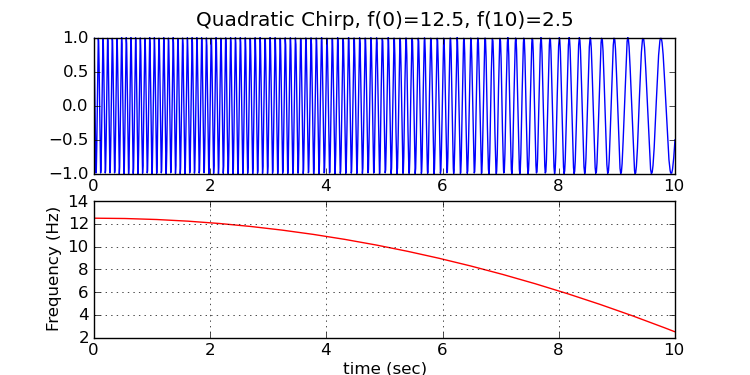Sample code using vertex_zero:

In :
t = np.linspace(0, 10, 5001)
w = chirp(t, f0=12.5, f1=2.5, t1=10, method='quadratic', vertex_zero=False)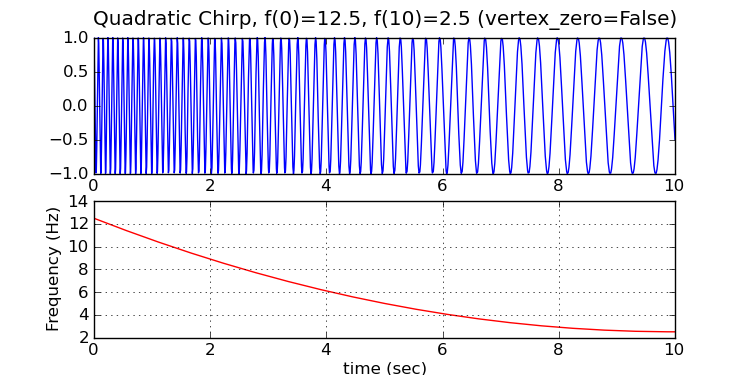## Logarithmic Chirp¶

Sample code:

In :
t = np.linspace(0, 10, 5001)
w = chirp(t, f0=12.5, f1=2.5, t1=10, method='logarithmic')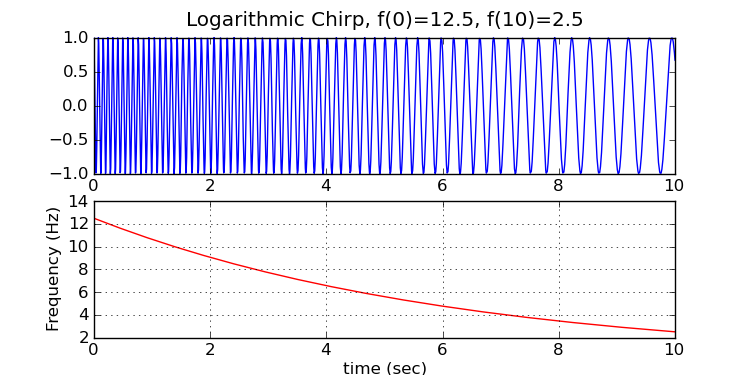## Hyperbolic Chirp¶

Sample code:

In :
t = np.linspace(0, 10, 5001)
w = chirp(t, f0=12.5, f1=2.5, t1=10, method='hyperbolic')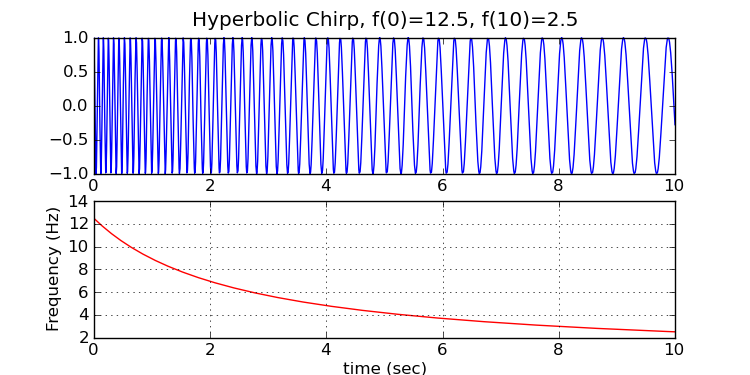## Sweep Poly¶

Sample code:

In :
p = np.poly1d([0.05, -0.75, 2.5, 5.0])
t = np.linspace(0, 10, 5001)
w = sweep_poly(t, p)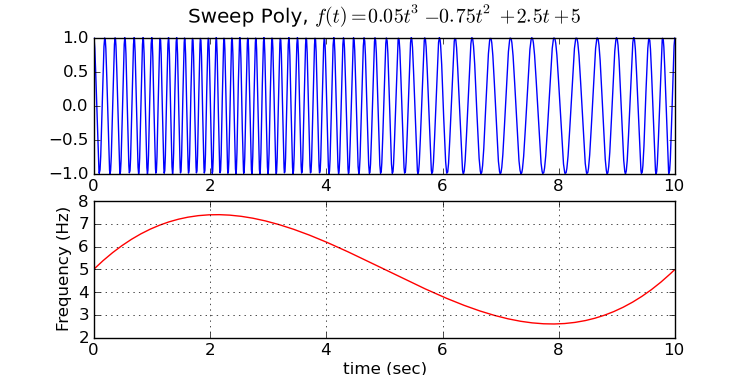The script that generated the plots is here

Section author: WarrenWeckesser

Attachments